• 一、创建策略和价值函数表达式(Policy and Value Function)：1、函数近似器(Function Approximation)2、Table Representations① 使用 rlTable创建 value table 或者 Q table ② 用 rlRepresentation为表格创建一个...


一、创建策略和价值函数表达式(Policy and Value Function)：1、函数近似器(Function Approximation)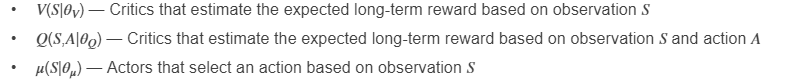2、Table Representations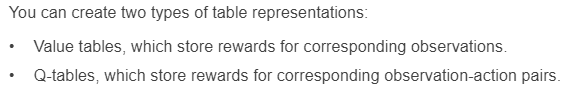① 使用 rlTable创建 value table 或者 Q table ② 用 rlRepresentation为表格创建一个表达式 (representation)③ rlRepresentationOptions配置学习率(learning rate)等参数。3、Deep Neural Network Representations (可以使用深度学习工具箱)    3.1 Network Input and Output Dimensions        ☆ actor 和 critic 网络的维度必须和环境的 observations 和 action 相匹配，获取环境(env)的action和observation的维度，使用 getActionInfo 和 getObservationInfo ，然后访问 dimension 属性。actInfo = getActionInfo(env);actDimensions = actInfo.Dimensions;obsInfo = getObservationInfo(env);obsDimensions = observationInfo.Dimensions;       ☆ 对于 AC 或者 PG等只需要 observations 作为输入的 critic network，输入层的维度必须匹配环境观测规范尺寸(environment observation specifications)，critic输出层的维度必须是一个标量值函数。        ☆ 对于DQN或者DDPG等需要 observations 和 actions作为输入的 critic networks，输入层的维度必须匹配environment observation and action specifications。Critic 输出层的维度也必须是一个标量值函数。        ☆ actor networks 输入层的维度必须匹配环境观测规范的维度            ①如果 actor 有离散的动作空间，他的输出维度必须等于离散动作的数量。            ②如果 actor 有连续的动作空间，它的输出维度必须是一个标量或者向量值，就像 observation specification中定义的那样。3.2 Build Deep Neural Network    下表列出强化学习常用的深度学习网络层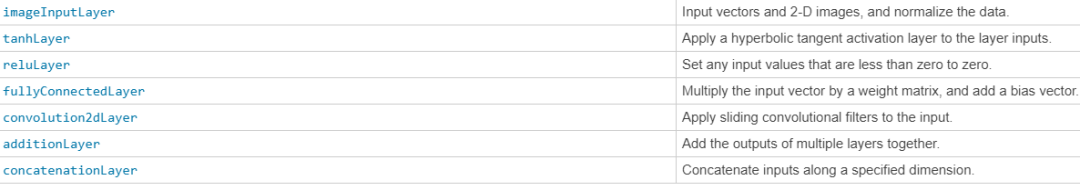☆ 注意：lstmLayer，bilstmLayer和 batchNormalizationLayer不支持强化学习。当然也可以自定义网络层。        scalingLayer线性缩放或者偏置输入数组。对于非线性层(tanhLayer 和 sigmoid)输出的缩放和移动(shifting)很有用        quadraticLayer从输入数组的元素构建二次单项式向量。当需要一个输出，它的输入是一些二次函数(例如LQR控制器),这个层会非常有用。    ☆ scalingLayer 和 quadraticLayer 自定义层不包含可调参数，在训练过程中不可改变。    ☆ 每一个输入路径(input path)通过 connectLayers 函数连接，构成一个深度神经网络。也可以用 Deep Network Designer app创建。对于 observation 和 action 输入路径，必须指定路径的第一个层是 imageInputLayer层。observationPath = [  imageInputLayer([4 1 1],'Normalization','none','Name','observation')  fullyConnectedLayer(24,'Name','CriticObsFC1')  reluLayer('Name','CriticRelu1')  fullyConnectedLayer(24,'Name','CriticObsFC2')];actionPath = [  imageInputLayer([1 1 1],'Normalization','none','Name','action')  fullyConnectedLayer(24,'Name','CriticActFC1')];commonPath = [  additionLayer(2,'Name','add')  reluLayer('Name','CriticCommonRelu')  fullyConnectedLayer(1,'Name','output')];criticNetwork = layerGraph(observationPath);criticNetwork = addLayers(criticNetwork,actionPath);criticNetwork = addLayers(criticNetwork,commonPath);criticNetwork = connectLayers(criticNetwork,'CriticObsFC2','add/in1');criticNetwork = connectLayers(criticNetwork,'CriticObsFC1','add/in2');    ☆ 通过 plot(criticNetwork) 查看深度神经网络的结构。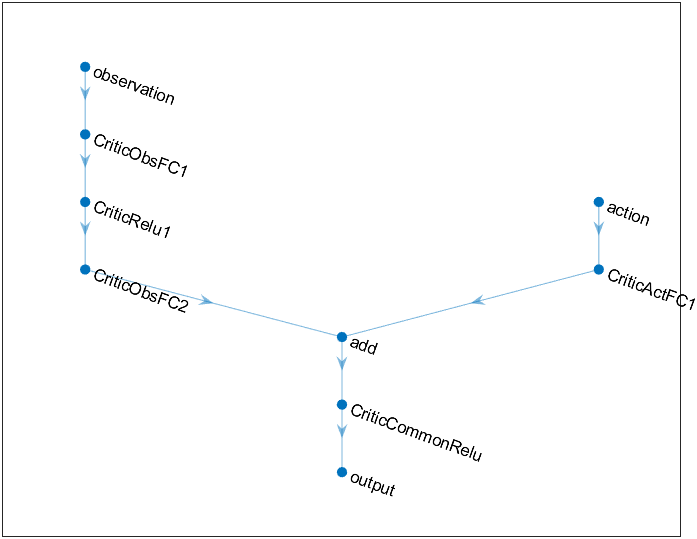☆对于 PG 和 AC 代理，深度神经网络 actor representation 的最终输出层是一个fullyConnectedLayer 和softmaxLayer。必须指定 fullyConnectedLayer ，因为当你省略softmaxLayer时，系统会自动添加一个 softmaxLayer。对于任何函数近似器最重要的是该函数能够逼近最优策略或者折扣价值函数(optimal policy or discounted value function)，也就是说能否正确获取 observation,action 和 reward 三个信号的特征(features)。    ☆当构建网络的时候必须考虑下面几点：        ① 对于连续的动作空间，用  tanhLayer 后面接 ScalingLayer 结构限制动作(bound actions)。        ② 带有 reluLayers 的深度密集网络(Deep dense network)能够很好的近似许多不同的函数。因此可以作为构建网络的第一选择。      ③ 当逼近强非线性(strong nonlinearities)系统或者带有代数约束(algebraic constraints)的系统，增加更多的层(more layers)往往好于增加每层输出的数量(increase number of outputs per layer)。增加更多的层提高指数搜索能力(exponential exploration)，增加每层的输出提高多项式探索能力(polynomial exploration)。        ④ 对于 on-policy 代理，例如 AC 和 PG ，如果网络非常大(例如网络具有两个隐藏层，每个隐藏层有32个节点，每个节点有上百个参数)parallel training 训练效率更高。On-policy parallel updates 假设每个 worker 更新网络的不同部分(例如探索 observation space 不同的区域)。如果网络比较小，worker 更新会互相关联，导致训练不稳定。3.3 Create and Configure Representation    ☆ 为深度神经网络创建 actor 或者 critic representation object，使用  rlRepresentation 函数。用  rlRepresentationOptions 配置学习率等参数。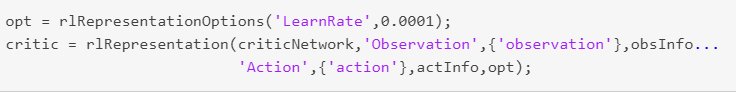☆ 当创建自己的深度神经网络并且配置 representation object，初始考虑使用下面的方法：        ① 最开始采用最小的网络和较大的学习率(0.01)。训练初始网络看一下是否代理很快收敛到很差的策略(poor policy)或者以随机的方式动作。如果出现这些问题，增加层或者增加输出的数量。你的目标是找到一个足够大的网络结构(而不是追求网络学习很快)，并且在初步训练后显示学习的迹象(奖励的改进轨迹)        ② 初始通过设置小的学习率使得网络训练缓慢。通过慢慢训练，可以检查代理是否在正确的轨迹上，这样可以判断网络结构是否满足问题的要求。对于复杂的问题，优秀的网络结构会使调整参数变得容易。    ☆ 当配置深度神经网络时考虑下面的技巧：        ① 对 DDPG和DQN要有耐心，因为他们可能在早期阶段一段时间内没有学到任何东西，他们通常在训练过程的早期表现出累积奖励的下降。最终，他们可以在头几千迭代(episode)之后表现出学习的迹象。        ② 对于具有 actor 和 critic 网络的代理，设置相同的初始学习率。对于一些问题，将 critic 的学习率设置的大一些可以改善学习效果。4 Specify Agent Representations     ☆ 当创建 actor 和 critic 表达式(representation)以后，可以用这些表达式创建一个强化学习代理。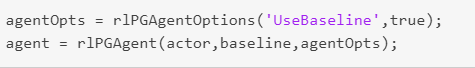☆ 可以通过  getActor 和 getCritic 命令获得已有代理的 actor and critic representations。也可以通过  setActor 和 setCritic 设置已存在代理的  actor and critic 。


展开全文• MATLAB提供了解决微分和积分微积分的各种方法，求解任何程度的微分方程和极限计算。可以轻松绘制复杂功能的图形，并通过求解原始功能以及其衍生来检查图形上的最大值，最小值和其他固定点。本章将介绍微积分问题。在...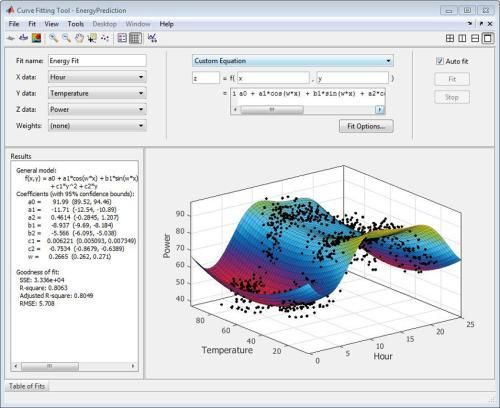MATLAB提供了解决微分和积分微积分的各种方法，求解任何程度的微分方程和极限计算。可以轻松绘制复杂功能的图形，并通过求解原始功能以及其衍生来检查图形上的最大值，最小值和其他固定点。本章将介绍微积分问题。在本章中，将讨论预演算法，即计算功能限制和验证限制属性。在下一章微分中，将计表达式的导数，并找到一个图的局部最大值和最小值。我们还将讨论求解微分方程。最后，在“整合/集成”一章中，我们将讨论积分微积分。计算极限MATLAB提供计算极限的limit函数。在其最基本的形式中，limit函数将表达式作为参数，并在独立变量为零时找到表达式的极限。例如，要计算函数f(x)=(x^3 + 5)/(x^4 + 7)的极限，因为x趋向于零。syms xlimit((x^3 + 5)/(x^4 + 7))MATLAB执行上面示例代码，得到以下结果 -Trial>> syms x limit((x^3 + 5)/(x^4 + 7)) ans = 5/7Shelllimit函数落在符号计算域; 需要使用syms函数来告诉MATLAB正在使用的符号变量。还可以计算函数的极限，因为变量趋向于除零之外的某个数字。要计算 -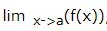可使用带有参数的limit命令。第一个是表达式，第二个是数字 - x表示接近，这里它是a。例如，要计算函数f(x)=(x-3)/(x-1)的极限，因为x倾向于1。limit((x - 3)/(x-1),1)MATLAB执行上面示例代码，得到以下结果 -ans = NaNShell下面再看另外一个例子，limit(x^2 + 5, 3)Shell执行上面示例代码，得到以下结果 -ans = 14Shell使用Octave计算极限以下是Octave版本的上述示例使用symbolic包，尝试执行并比较结果 -pkg load symbolic symbols x=sym("x");subs((x^3+5)/(x^4+7),x,0)MATLAB执行上面示例代码，得到以下结果 -ans = 0.7142857142857142857Shell验证极限的基本属性代数极限定理提供了极限的一些基本属性。这些属性如下 -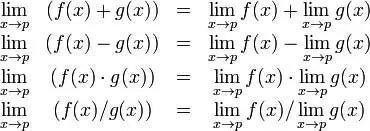下面来考虑两个函数 -f(x) = (3x + 5)/(x - 3) g(x) = x^2 + 1.下面计算函数的极限，这两个函数的x趋向于5，并使用这两个函数和MATLAB验证极限的基本属性。例子创建脚本文件并在其中键入以下代码 -syms x f = (3*x + 5)/(x-3);g = x^2 + 1;l1 = limit(f, 4)l2 = limit (g, 4)lAdd = limit(f + g, 4)lSub = limit(f - g, 4)lMult = limit(f*g, 4)lDiv = limit (f/g, 4)MATLAB执行上面示例代码，得到以下结果 -l1 = 17 l2 = 17 lAdd = 34 lSub = 0 lMult = 289 lDiv = 1Shell使用Octave验证极限的基本属性以下是Octave版本的上述示例使用symbolic包，尝试执行并比较结果 -pkg load symbolic symbols x = sym("x");f = (3*x + 5)/(x-3);g = x^2 + 1;l1=subs(f, x, 4)l2 = subs (g, x, 4)lAdd = subs (f+g, x, 4)lSub = subs (f-g, x, 4)lMult = subs (f*g, x, 4)lDiv = subs (f/g, x, 4)MATLAB执行上面示例代码，得到以下结果 -l1 = 17.0 l2 = 17.0 lAdd = 34.0 lSub = 0.0 lMult = 289.0 lDiv = 1.0Shell左右边界极限当函数对变量的某个特定值具有不连续性时，该点不存在极限。 换句话说，当x = a时，函数f(x)的极限具有不连续性，当x的值从左侧接近x时，x的值不等于x从右侧接近的极限值。对于x <a的值，左极限被定义为x - > a的极限，从左侧即x接近a。 对于x> a的值，右极限被定义为x - > a的极限，从右边，即x接近a。 当左极限和右极限不相等时，极限不存在。下面来看看一个函数 -f(x) = (x - 3)/|x - 3|下面将显示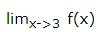不存在。MATLAB帮助我们以两种方式说明事实 -通过绘制函数图并显示不连续性。通过计算极限并显示两者都不同。通过将字符串“left”和“right”作为最后一个参数传递给limit命令来计算左侧和右侧的极限。例子创建脚本文件并在其中键入以下代码 -f = (x - 3)/abs(x-3);ezplot(f,[-1,5])l = limit(f,x,3,'left')r = limit(f,x,3,'right')MATLAB执行上面示例代码，得到以下结果 -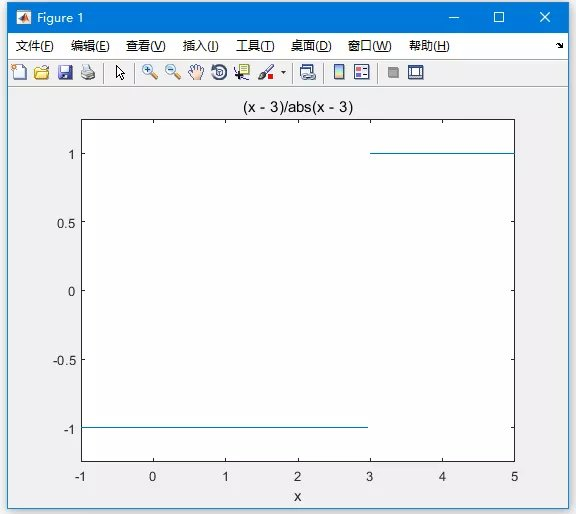显示以下输出结果 -Trial>> Trial>> f = (x - 3)/abs(x-3); ezplot(f,[-1,5]) l = limit(f,x,3,'left') r = limit(f,x,3,'right') l = -1 r = 1ShellMATLAB提供用于计算符号导数的diff命令。 以最简单的形式，将要微分的功能传递给diff命令作为参数。例如，计算函数的导数的方程式 -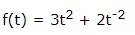例子创建脚本文件并在其中键入以下代码 -syms t f = 3*t^2 + 2*t^(-2);diff(f)MATLAB执行上面示例代码，得到以下结果 -Trial>> syms t f = 3*t^2 + 2*t^(-2); diff(f) ans = 6*t - 4/t^3Shell以下是使用Octave 计算的写法 -pkg load symbolic symbols t = sym("t");f = 3*t^2 + 2*t^(-2);differentiate(f,t)MATLAB执行上面示例代码，得到以下结果 -ans = 6*t - 4/t^3Shell基本微分规则的验证下面简要说明微分规则的各种方程或规则，并验证这些规则。 为此，我们将写一个第一阶导数f'(x)和二阶导数f“(x)。以下是微分的规则 -规则 - 1对于任何函数f和g，任何实数a和b是函数的导数：h(x) = af(x) + bg(x)相对于x，由h’(x) = af’(x) + bg’(x)给出。规则 - 2sum和subtraction规则表述为：如果f和g是两个函数，则f'和g'分别是它们的导数，如下 -(f + g)' = f' + g' (f - g)' = f' - g'规则 - 3product规则表述为：如果f和g是两个函数，则f'和g'分别是它们的导数，如下 -(f.g)' = f'.g + g'.f规则 - 4quotient规则表明，如果f和g是两个函数，则f'和g'分别是它们的导数，那么 -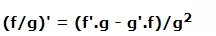规则 - 5多项式或基本次幂规则表述为：如果y = f(x)= x^n，则 -这个规则的直接结果是任何常数的导数为零，即如果y = k，那么为任何常数 -f' = 0规则 - 5chain规则表述为 - 相对于x的函数h(x)= f(g(x))的函数的导数是 -h'(x)= f'(g(x)).g'(x)MATLAB例子创建脚本文件并在其中键入以下代码 -syms x syms t f = (x + 2)*(x^2 + 3)der1 = diff(f)f = (t^2 + 3)*(sqrt(t) + t^3)der2 = diff(f)f = (x^2 - 2*x + 1)*(3*x^3 - 5*x^2 + 2)der3 = diff(f)f = (2*x^2 + 3*x)/(x^3 + 1)der4 = diff(f)f = (x^2 + 1)^17der5 = diff(f)f = (t^3 + 3* t^2 + 5*t -9)^(-6)der6 = diff(f)MATLAB执行上面示例代码，得到 以下结果 -f = (x^2 + 3)*(x + 2) der1 = 2*x*(x + 2) + x^2 + 3 f = (t^(1/2) + t^3)*(t^2 + 3) der2 = (t^2 + 3)*(3*t^2 + 1/(2*t^(1/2))) + 2*t*(t^(1/2) + t^3) f = (x^2 - 2*x + 1)*(3*x^3 - 5*x^2 + 2) der3 = (2*x - 2)*(3*x^3 - 5*x^2 + 2) - (- 9*x^2 + 10*x)*(x^2 - 2*x + 1) f = (2*x^2 + 3*x)/(x^3 + 1) der4 = (4*x + 3)/(x^3 + 1) - (3*x^2*(2*x^2 + 3*x))/(x^3 + 1)^2 f = (x^2 + 1)^17 der5 = 34*x*(x^2 + 1)^16 f = 1/(t^3 + 3*t^2 + 5*t - 9)^6 der6 = -(6*(3*t^2 + 6*t + 5))/(t^3 + 3*t^2 + 5*t - 9)^7Shell以下是对上面示例的Octave写法 -pkg load symbolic symbols x=sym("x");t=sym("t");f = (x + 2)*(x^2 + 3) der1 = differentiate(f,x) f = (t^2 + 3)*(t^(1/2) + t^3) der2 = differentiate(f,t) f = (x^2 - 2*x + 1)*(3*x^3 - 5*x^2 + 2) der3 = differentiate(f,x) f = (2*x^2 + 3*x)/(x^3 + 1) der4 = differentiate(f,x) f = (x^2 + 1)^17 der5 = differentiate(f,x) f = (t^3 + 3* t^2 + 5*t -9)^(-6) der6 = differentiate(f,t)MATLAB指数，对数和三角函数的导数下表提供了常用指数，对数和三角函数的导数，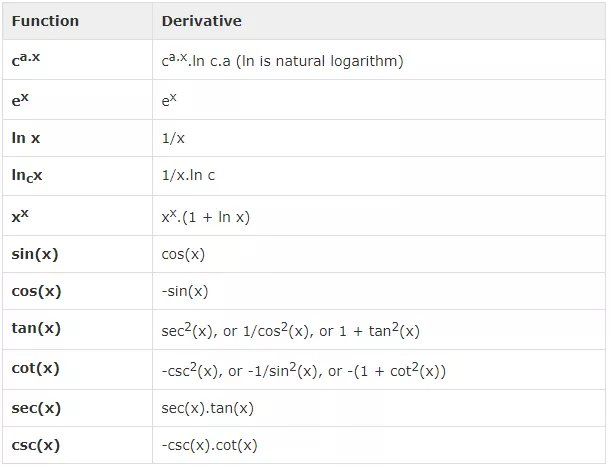例子创建脚本文件并在其中键入以下代码 -syms x y = exp(x)diff(y)y = x^9diff(y)y = sin(x)diff(y)y = tan(x)diff(y)y = cos(x)diff(y)y = log(x)diff(y)y = log10(x)diff(y)y = sin(x)^2diff(y)y = cos(3*x^2 + 2*x + 1)diff(y)y = exp(x)/sin(x)diff(y)MATLAB执行上面示例代码，得到以下结果 -y = exp(x) ans = exp(x) y = x^9 ans = 9*x^8 y = sin(x) ans = cos(x) y = tan(x) ans = tan(x)^2 + 1 y = cos(x) ans = -sin(x) y = log(x) ans = 1/x y = log(x)/log(10) ans = 1/(x*log(10)) y = sin(x)^2  ans = 2*cos(x)*sin(x) y = cos(3*x^2 + 2*x + 1) ans = -sin(3*x^2 + 2*x + 1)*(6*x + 2) y = exp(x)/sin(x) ans = exp(x)/sin(x) - (exp(x)*cos(x))/sin(x)^2Shell以下代码是上面代码的Octave写法 -pkg load symbolic symbols x = sym("x"); y = Exp(x) differentiate(y,x) y = x^9 differentiate(y,x) y = Sin(x) differentiate(y,x) y = Tan(x) differentiate(y,x) y = Cos(x) differentiate(y,x) y = Log(x) differentiate(y,x) % symbolic packages does not have this support %y = Log10(x) %differentiate(y,x) y = Sin(x)^2 differentiate(y,x) y = Cos(3*x^2 + 2*x + 1) differentiate(y,x) y = Exp(x)/Sin(x) differentiate(y,x)Shell计算高阶导数要计算函数f的较高导数，可使用diff(f，n)。计算函数的二阶导数公式为 -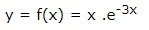f = x*exp(-3*x);diff(f, 2)MATLABMATLAB执行上面代码将返回以下结果 -ans = 9*x*exp(-3*x) - 6*exp(-3*x)Shell以下是使用Octave重写上面示例，代码如下 -pkg load symbolic symbols x = sym("x");f = x*Exp(-3*x);differentiate(f, x, 2)MATLAB例子在这个例子中，要解决一个问题。由给定函数y = f(x)= 3sin(x)+ 7cos(5x)，来找出方程f“+ f = -5cos(2x)是否成立。创建脚本文件并在其中键入以下代码 -syms x y = 3*sin(x)+7*cos(5*x);  % defining the functionlhs = diff(y,2)+y;        %evaluting the lhs of the equationrhs = -5*cos(2*x);        %rhs of the equationif(isequal(lhs,rhs))    disp('Yes, the equation holds true');else    disp('No, the equation does not hold true');enddisp('Value of LHS is: '), disp(lhs);MATLAB运行文件时，会显示以下结果 -No, the equation does not hold true Value of LHS is: -168*cos(5*x)Shell以上是上面示例的Octave写法 -pkg load symbolic symbols x = sym("x");y = 3*Sin(x)+7*Cos(5*x);           % defining the functionlhs = differentiate(y, x, 2) + y;  %evaluting the lhs of the equationrhs = -5*Cos(2*x);                 %rhs of the equationif(lhs == rhs)    disp('Yes, the equation holds true');else    disp('No, the equation does not hold true');enddisp('Value of LHS is: '), disp(lhs);MATLAB查找曲线的最大和最小值如果正在搜索图形的局部最大值和最小值，基本上是在特定地点的函数图上或符号变量的特定值范围内查找最高点或最低点。对于函数y = f(x)，图形具有零斜率的图上的点称为固定点。 换句话说，固定点是f'(x)= 0。要找到微分的函数的固定点，需要将导数设置为零并求解方程。示例要找到函数f(x)= 2x3 + 3x2 - 12x + 17的固定点可参考以下步骤 -首先输入函数并绘制图，代码如下 -syms x y = 2*x^3 + 3*x^2 - 12*x + 17;  % defining the functionezplot(y)MATLAB执行上面示例代码，得到以下结果 -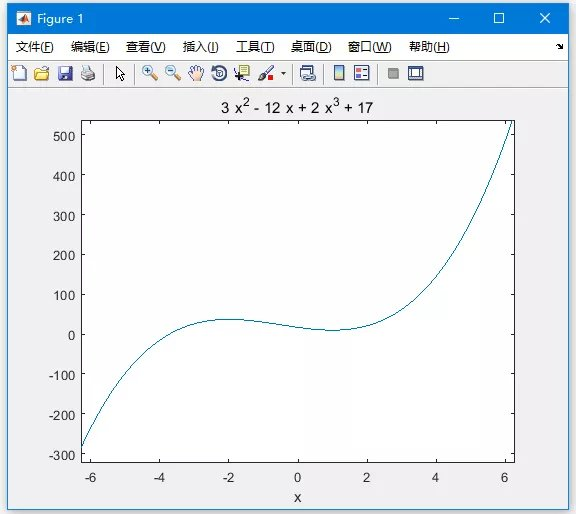以上是上面示例的Octave写法 -pkg load symbolic symbols x = sym('x');y = inline("2*x^3 + 3*x^2 - 12*x + 17");ezplot(y)print -deps graph.epsMATLAB我们的目标是在图上找到一些局部最大值和最小值，假设要找到图中间隔在[-2,2]的局部最大值和最小值。参考以下示例代码 -syms x y = 2*x^3 + 3*x^2 - 12*x + 17;  % defining the functionezplot(y, [-2, 2])MATLAB执行上面示例代码，得到以下结果 -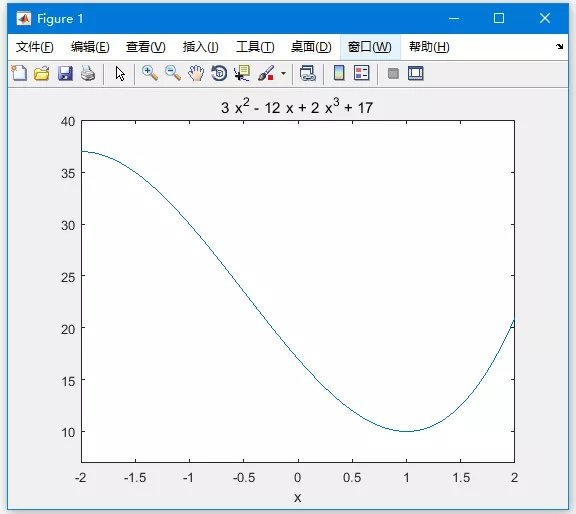以下是上面示例的Octave写法 -pkg load symbolic symbols x = sym('x');y = inline("2*x^3 + 3*x^2 - 12*x + 17");ezplot(y, [-2, 2])print -deps graph.epsMATLAB接下来，需要计算导数。g = diff(y)MATLABMATLAB执行代码并返回以下结果 -g = 6*x^2 + 6*x - 12Shell以下是上面示例的Octave写法 -pkg load symbolic symbols x = sym("x");y = 2*x^3 + 3*x^2 - 12*x + 17;g = differentiate(y,x)MATLAB接下来求解导数函数g，得到它变为零的值。s = solve(g)MATLABMATLAB执行代码并返回以下结果 -s =     1    -2Shell以下是上面示例的Octave写法 -pkg load symbolic symbols x = sym("x");y = 2*x^3 + 3*x^2 - 12*x + 17;g = differentiate(y,x)roots([6, 6, -12])MATLAB这与我们设想情节一致。 因此，要评估临界点x = 1，-2处的函数f。可以使用subs命令替换符号函数中的值。subs(y, 1), subs(y, -2)MATLABMATLAB执行代码并返回以下结果 -ans = 10 ans = 37Shell以下是上面示例的Octave写法 -pkg load symbolic symbols x = sym("x");y = 2*x^3 + 3*x^2 - 12*x + 17;g = differentiate(y,x)roots([6, 6, -12])subs(y, x, 1), subs(y, x, -2)MATLAB因此，在间隔[-2,2]中函数f(x)= 2x^3 + 3x^2 - 12x + 17的最小值和最大值分别为10和37。求解微分方程MATLAB提供了用于求解微分方程的dsolve命令。找到单个方程的解的最基本的dsolve命令形式是 -dsolve('eqn')MATLAB其中eqn是用于输入方程式的文本串。它返回一个符号解，其中包含一组任意常量，MATLAB标记C1，C2等等。还可以为问题指定初始和边界条件，以逗号分隔的列表遵循以下公式：dsolve('eqn','cond1', 'cond2',…)为了使用dsolve命令，导数用D表示。例如，像f'(t)= -2 * f + cost(t)这样的等式输入为 -'Df = -2*f + cos(t)'较高阶导数由D导数的顺序表示。例如，方程f"(x) + 2f'(x) = 5sin3x应输入为 -'D2y + 2Dy = 5*sin(3*x)'下面来看一个一阶微分方程的简单例子：y'= 5y。s = dsolve('Dy = 5*y')MATLAB执行代码并返回以下结果 -s = C2*exp(5*t)Shell再来一个二阶微分方程的例子：y“-y = 0，y(0)= -1，y'(0)= 2。dsolve('D2y - y = 0','y(0) = -1','Dy(0) = 2')MATLABMATLAB执行代码并返回以下结果 -ans = exp(t)/2 - (3*exp(-t))/2Shell
展开全文• MATLAB中绘图首先需要设定x的范围，一般使用冒号来创建向量。例如 x = 0:pi/100:pi; &x 为0到pi之间以pi/100为步长的向量。(1) plot()二维图形的绘制要求：x和y需要有相同长度的向量调用形式：plot(x...点击上方蓝字  关注我们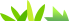MATLAB有很强的绘图功能，我们可以通过简单的代码画出一些直观而漂亮的代码。今天，我们一起来学习基本绘图常用函数。在MATLAB中绘图首先需要设定x的范围，一般使用冒号来创建向量。例如 x = 0:pi/100:pi; &x 为0到pi之间以pi/100为步长的向量。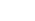(1) plot()二维图形的绘制要求：x和y需要有相同长度的向量调用形式：plot(x)  :绘制出以x为纵坐标值的折线图，横轴值默认为1、2、3……。plot(x,y): 绘制出以x向量为自变量的y函数在给出x的范围内的图像。plot(y):  在给定的x范围内绘制出函数y的图像。plot(x1,y1,……xn,yn):绘制多个X、Y对组的图，注意所有线条都使用相同的坐标区。(x1,x2,x3……xn都应相同)plot(x,y'……') 此类形式方便对线形颜色等属性的修改。>> x = 0:pi/100:2*pi;>> y = sin(x);>> y1 = cos(x);>> plot(x);>> plot(x,y);>> plot(x,y,x,y1);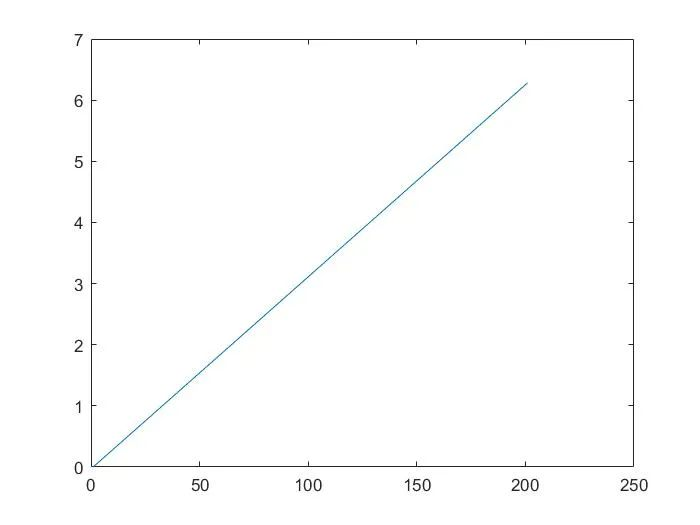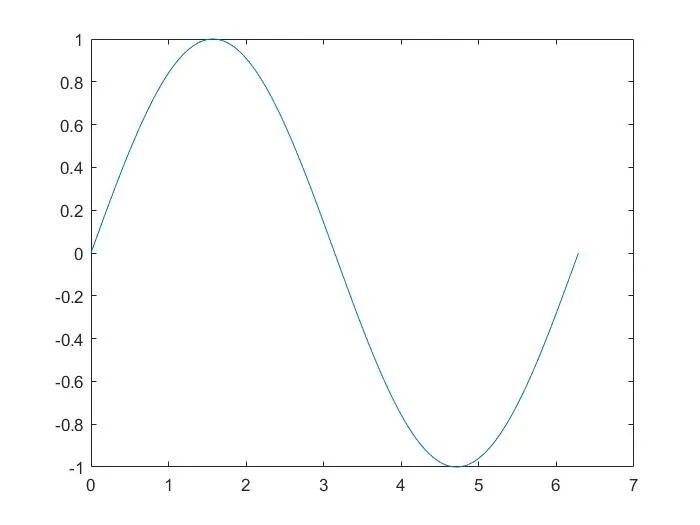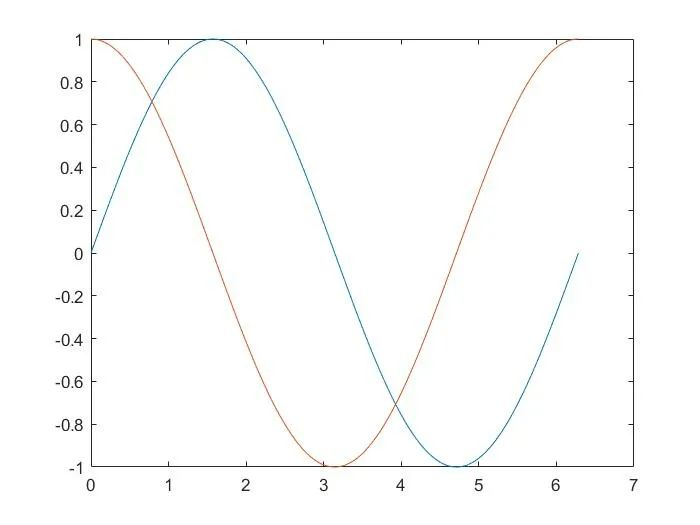(2) fplot()符号函数的绘制函数fplot可以根据函数的表达式自动调整自变量的范围，无须给函数赋值，直接生成能反应函数变化规律的图形，调用格式:fplot(y) : 在默认区间[-5 5](对于x)绘制由函数y = f(x)定义的曲线。fplot(y,xinterval):将在指定区间绘图。将区间指定为[xmin xmax]形式的二元素向量。fplot(funx,funy):在默认区间[-5 5](对于t)绘制由x = funx(t)和y = funy(t)定义的曲线。fplot(funx,funy,interval):将在指定区间绘图。将区间指定为[tmin tmax]形式的二元素向量。fplot(  ,linespace)   linespace 为线形，次函数可以修改所绘制图像曲线的线形。>> fplot(@(x) sin(x))%绘制匿名函数，使用句柄。>> fplot(@(t)t.*sin(t),@(t)t.*cos(t))%绘制参数方程，x =t*sin(t);y =t*cos(t);&其它例子大致相同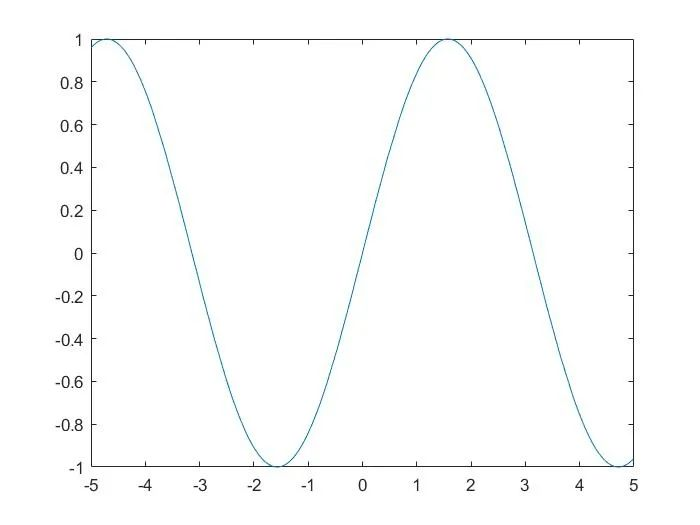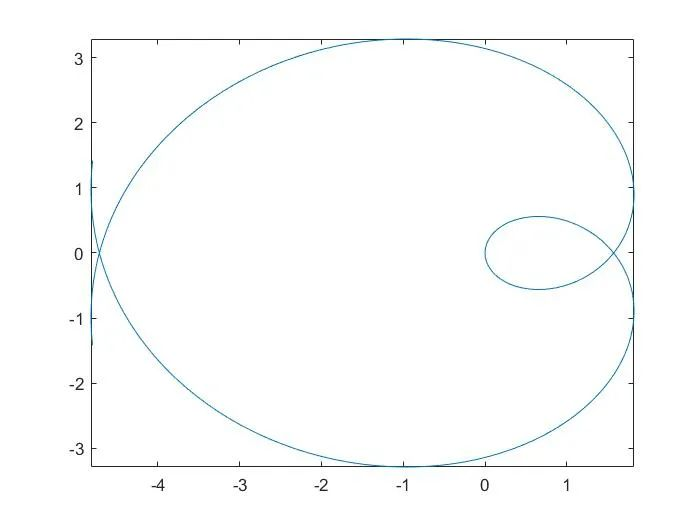注意该fplot函数中的y以及funx,funy所代表的函数，它们一般是以句柄的形式给出。关于MATLAB中句柄的建立以及使用请参考：MATLAB中函数句柄@的作用及介绍：https://link.zhihu.com/?target=https%3A//www.cnblogs.com/alexanderkun/p/4617107.html                                                    (3)plotyy()双Y轴图形绘制该函数可以在一个图中创建有两个Y轴的图形。调用格式：plotyy(x1,y1,x2,y2):  绘制Y1对X1的图，在左侧显示y轴标签，并同时绘制Y2对X2的图，在右侧显示y轴标签。plotyy(x1,y1,x2,y2,function):使用指定的绘图函数生成图形。常见的function函数有plot、semilogx(半对数图中按照x轴的对数刻度绘制数据。)、semilogy(半对数图中使用y轴的对数刻度绘制数据。)、loglog(双对数图)、stem(绘制离散序列数据)详细参见文末链接。plotyy(x1,y1,x2,y2,'function1','function2'):使用function1(X1,Y1)绘制左轴的数据，使用function2(X2,Y2)绘制右轴的数据。>> x = 0:pi/100:2*pi;>> y1 = sin(x);>> y2 = cos(x);>> plotyy(x,y1,x,y2)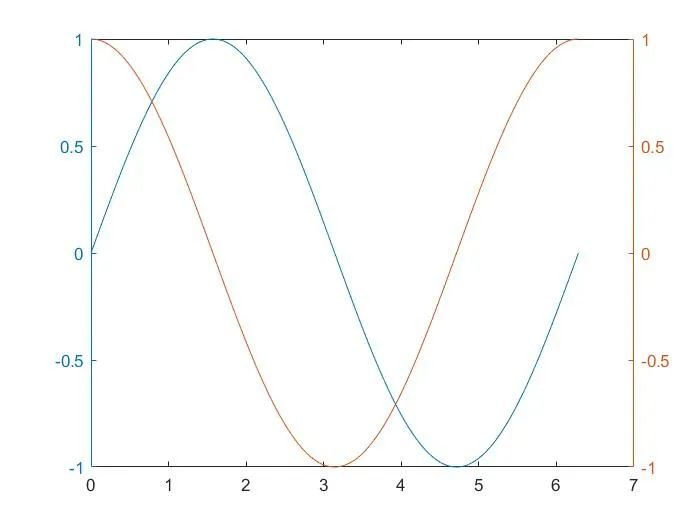>> x = [0,10^6,7]>> y3 = x;>> y4 = exp(x);>> plotyy(x,y3,x,y4,'semilogx')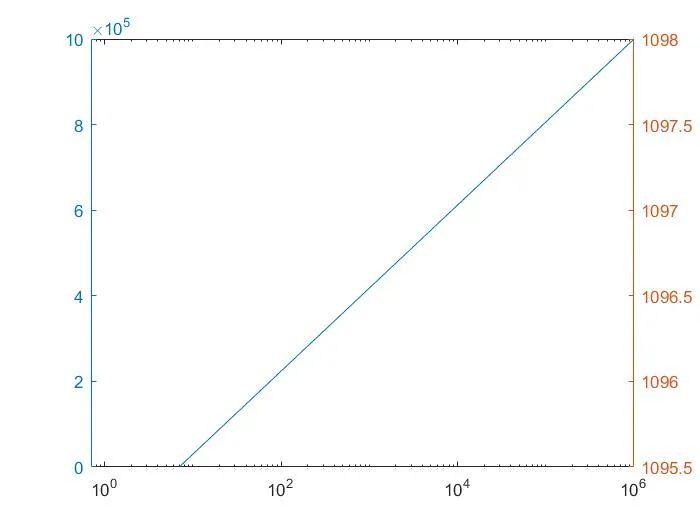(4) ezplot() 符号函数的绘制ezplot()函数与fplot函数的功能基本相似，可以方便地绘制表达式或函数的图形。与fplot函数不同的是函数的表达式显示在图形的上方，同时对坐标轴可以不加任何限制作图。调用格式：ezplot(fun):  绘制表达式fun(x)在默认定义域 -2π <x< 2π 上的图形，其中fun(x)仅是x的显函数。ezplot(fun,[xmin,xmax]):绘制fun(x)在以下域上的图形：xmin<x<xmax。对于隐函数，fun2(x,y)：ezplot(fun2) 在默认域 -2π x < 2π 和 -2π y < 2π 中绘制 fun2(x,y) = 0。ezplot(fun2,[xymin,xymax]) 在 xymin x xymax 和 xymin y xymax 域中绘制 fun2(x,y) = 0。ezplot(fun2,[xmin,xmax,ymin,ymax]) 在 xmin x xmax 和 ymin y ymax 域中绘制 fun2(x,y) = 0。>> subplot(221)>> ezplot('x^2+sin(x)');>> subplot(222)>> ezplot('x^2+sin(x)',[-2,8])>> subplot(223)    %subplot()函数可以在一张图幅上画出多个图像，如下图所示。>> subplot(2,2,[3,4])>> ezplot('x^2+sin(x)',[-2,5,-1,20])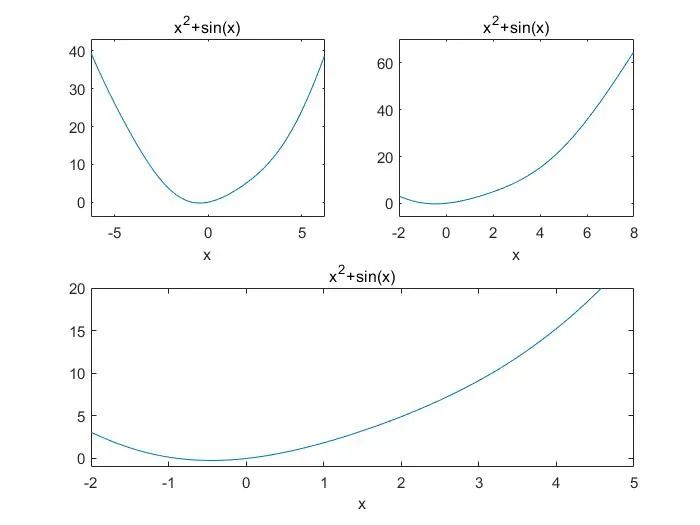END对于半对数图，双对数图参考以下链接：半对数图—MATLAB semilogx:https://link.zhihu.com/?target=https%3A//ww2.mathworks.cn/help/matlab/ref/semilogx.html%3Fs_tid%3Ddoc_ta半对数图—MATLAB semilogy:https://link.zhihu.com/?target=https%3A//ww2.mathworks.cn/help/matlab/ref/semilogy.html%3Fs_tid%3Ddoc_ta双对数刻度图—MATLAB loglog:https://link.zhihu.com/?target=https%3A//ww2.mathworks.cn/help/matlab/ref/loglog.html%3Fs_tid%3Ddoc_ta绘制离散序列数据—MATLAB stem:https://link.zhihu.com/?target=https%3A//ww2.mathworks.cn/help/matlab/ref/stem.html%3Fs_tid%3Ddoc_ta本文参考：清华大学出版社《MATLAB基础与可视化编程》MATLAB MathWorks官网本文仅供学习交流所用，由于能力不足，编写不当之处望批评指正。关于MATLAB的学习：大家可以关注我们的知乎专栏——数据可视化和数据分析中matlab的使用：https://zhuanlan.zhihu.com/c_1131568134137692160欢迎大家加入我们的MATLAB学习交流群：953314432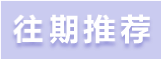MATLAB界面介绍及学习方法(附有视频讲解)数学建模与MATLAB–整数规划(附有代码讲解)数学建模与MATLAB--非线性规划数学建模与MATLAB——动态规划扫码关注我们了解更多精彩出品：Asoul水云天课堂工作室

展开全文• 如何使用产品标量值创建向量函数句柄(匿名函数),然后绘制结果.显然,我想绘制这个公式Let f(x,ym)= (1-exp(x-ym)/h); andiX(x)=1/6.f(x,ym(1))+7/6.f(x,ym(2))+25/6.f(x,ym(3))+43/6.f(x,ym(4));iY(x)=1/5.f(x,ym(1))...
如何使用产品标量值创建向量函数句柄(匿名函数),然后绘制结果.显然,我想绘制这个公式Let f(x,ym)= (1-exp(x-ym)/h); andiX(x)=1/6.f(x,ym(1))+7/6.f(x,ym(2))+25/6.f(x,ym(3))+43/6.f(x,ym(4));iY(x)=1/5.f(x,ym(1))+2/5.f(x,ym(2))+14/5.f(x,ym(3))+4/5.f(x,ym(4));这个代码没有循环但是给我：”使用绘图时出错转换从sym加倍是不可能的”.clear all;clc;a=0;b=2;n=3;h=(b-a)/n;ym=a:h:b;X=[ 1/6, 7/6, 25/6,43/6];  % DataY=[ 1/5, 2/5, 14/5,14/5];f=@(x,ym) (1-exp(x-ym)/h);% with symssyms x;iX=f(x,ym(1:length(ym))).*X; % result iX= [f.1/6 , f.7/6 , f.25/6 , f.43/6]iY=f(x,ym(1:length(ym))).*Y;iFx= sum(iX);iFy=sum(iY);   % result  iFx= f.1/6 + f.7/6 + f.25/6 + f.43/6x=ym;plot(x,iFx,'r+');hold on;plot(x,iFy,'og');此外,我厌倦了使用具有循环条件的替代代码,但错误仍然存​​在,因为无法从sym转换为逻辑.% clear all;clc% a=0;b=2;n=3;h=(b-a)/n;ym=a:h:b;% %% X=[ 1/6, 7/6, 25/6,43/6]; % Data% Y=[ 1/5, 2/5, 14/5,4/5];% %% f=@(x,ym) (1-exp(x-ym)/h);% %% syms x  %double(subs(x))% iX=cell(1,length(ym));iY=cell(1,length(ym)); %  cell, iX{k} and iY{k}% % iX=zeros(1,length(ym));iY=zeros(1,length(ym));% for k=1:length(ym)% %          iX(k) =   f(x,ym(k)).*X(k);% %          iY(k) =   f(x,ym(k)).*Y(k);%            iX{k} = @(x,k)  f(x,ym(k)).*X(k);%            iY{k} = @(x,k)  f(x,ym(k)).*Y(k);%            iX(x,k);% end% whos% x=ym;% plot(x,iX{:},'r:');hold on;plot(x,iY{:},'b--');拜托,我该怎么办呢？
展开全文• 绘制表达式函数 二、语法 1.fplot(f) 在默认区间[-5 5]（对于x）绘制由函数y = f(x)定义的曲线。 2.fplot(f,xinterval) 将在指定区间绘图。将区间指定为[xmin xmax]形式的二元素向量。 3.fplot(funx,funy) ...
• >> ht = matlabFunction(sin(r)/r) ht = @(x,y)sin(sqrt(x.^2+y.^2)).*1./sqrt(x.^2+y.^2) Option #2: Generate a function by hand Since you've already written a set of symbolic equations, you can simply ...
• odeplot ODE 输出函数的时间轨迹图 odeprint 在 Matlab 指令窗显示结果 odeset 创建或改写 ODE 选项构架参数值 ones 全 1 数组 optimset 创建或改写优化泛函指令的选项......matlab函数大全(非常实用)_数学_自然科学...
• 引言.啥是正则表达式？正则表达式是干啥的？我理解就和我们在word或者其他编辑软件里点的查找、替换的作用是差不多的，不过功能要强大的多，...matlab提供的正则表达式函数有三个：regexp——用于对字符串进行查找，...
• 引言.啥是正则表达式？正则表达式是干啥的？ 我理解就和我们在word... matlab提供的正则表达式函数有三个： regexp——用于对字符串进行查找，大小写敏感； regexpi——用于对字符串进行查找token c
• MATLAB符号运算处理的主要对象是符号或符号表达式，为此要使用一种新的数据类型---符号变量。符号表达式创建可由符号''或sym函数来完成。
• 向各位求教一下 求教一下各位大神，为什么不能生成相应的txt Q-Q QAQ
• MATLAB函数函数是一组语句一起执行任务。在MATLAB中，函数定义在单独的文件。文件函数的文件名应该是相同的。函数操作在自己的工作空间，它也被称为本地工作区，独立的工作区，在 MATLAB命令提示符访问，这就是所谓...
• 内容导航：Q1：matlab怎么建立自定义函数自定义函数的格式：function 函数名(参数)函数表达式end并保存为 函数名.m 的文件(函数名由英文和数值字组成)下面以标准的正态分布函数为例，来建立其自定义函数。...
• In JavaScript, we have three ways to write any functions: function declaration, ... 在JavaScript中，我们可以通过三种方式编写任何函数:函数声明，函数表达式和箭头函数。 But I focused on the function ...python javascript c++ sql
• 到本节为止，我们已经看到，所有的例子 MATLAB 方式工作...对于因式分解以及简化代数表达式，我们也会进=进行接触。在MATLAB解决基本的代数方程组MATLAB 中使用 solve命令求解代数方程组。在其最简单的形式，solve...
• 目录一、建立文件二、函数调用二、函数句柄三、匿名函数想查找相关内容也可在页面按ctrl+F搜索文字一、建立文件新建脚本，保存后可运行，后缀为.m 二、函数调用创建ffib.m文件，编写求Fibonacci数列的函数function...
• Matlab构建匿名函数（@(var1,...)表达式）。
• 以下汇总了几种matlab创建网格的几个函数，供大家参考~~~~ 先点赞再看，不然都是流氓 目录linspace：生成线性间距向量语法说明示例等间距数字向量包含指定数量的值的向量由均匀分布的复数组成的向量输入参数x1,x2...
• http://hi.baidu.com/daihaipengdhp/blog/item/16dbf417a950650dc93d6db4.html 引言.啥是正则表达式？... 书上的定义差不多是这样的：正则表达式就是一个表达式（也是一串字符），它定义了某种...
• 函数定义格式： function 变量名 = 函数名（n个参数） 描述语句   举例： function y = sinfun1(M) %函数功能和参数作用 x = 0 : M-1; for k = 1 : numel(x)  y(k) = sin(x(k) / (100 * pi)); end  注意：...
• matlab中如何实现符号变量替换符号表达式 , matlab 表达式中用的符号变量与算法中的符号变量不同(在matlab中如何实现符号变量替换符号表达式 , matlab 表达式中用的符号变量与算法中的符号变量不同(变量有多个......
• 一旦创建了一个符号表达式，或许想以某些方式改变它；也许希望提取表达式的一部分，合并两个表达式或求得表达的数值。有许多符号工具可以帮助完成这些任务。 　所有符号函数(很少特殊例外的情况，讨论于后)作用到...
• Matlab符号表达式的用法（1） 符号表达式 Help Search: Symbolic MathToolbox 第一节 包括的内容有微积分、线性代数、化简代数表达式、方程求解、特殊的数学函数、变量精度算法和数学变换 微积分：微分、积分...
• 一、课程目的初步学会使用MATLAB软件，掌握MATLAB基本的函数的使用，熟练MATLAB编程，以便为今后数字信号的处理打下基础。二、课程内容实验一 MATLAB语言基础实验目的：基本掌握 MATLAB 向量、矩阵、数组的生成及其...
• Matlab 正则表达式Matlab中支持函数字符元字符特殊字符限定符新的改变功能快捷键合理的创建标题，有助于目录的生成如何改变文本的样式插入链接与图片如何插入一段漂亮的代码片生成一个适合你的列表创建一个表格设定...
• ## MATLAB匿名函数

千次阅读 2017-09-19 22:31:51
匿名函数的基本定义fhandle=@(arglist)expr其中，expr是具体的函数表达式，arglist是指定的函数自变量。具体请见下面示例f=@(x)x.^2; >> fx=f(1:10) fx = 1 4 9 16 25 36 49 64 81 100 >> g=@(x,y)x.^2+y.^2; >> gxy...
• 如果你想知道系统辨识能做什么，可以看我之前写过的文章，通过系统辨识出传递函数，来精准调节PID。 龙胆也老师：matlab系统辨识+调PID，全网最完整步骤（v-rep仿真）​zhuanlan.zhihu.com系统辨识--入门前言数学......

# matlab创建函数表达式matlab 订阅